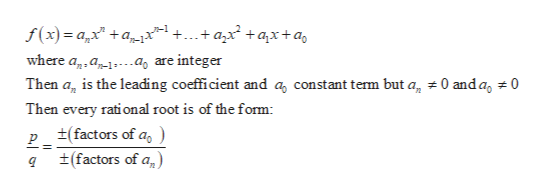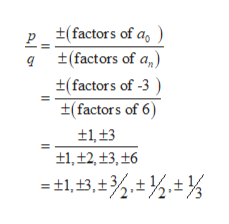# Give all possible rational zeros for the following polynomial. p(x)= 6x3 + 24x2 + 2x - 3

Question
99 views

Give all possible rational zeros for the following polynomial.
p(x)= 6x3 + 24x2 + 2x - 3

check_circle

Step 1

Consider the polynomial function p(x) = 6x3 + 24x2 + 2x – 3. The leading coefficient is 6 and constant term is -3.

To find all possible zero of the polynomial need to apply Rational Root Test to the polynomial. According to Rational Root Test:

A polynomial of the formhelp_outlineImage Transcriptionclosef(x)=ax +a_zx^l ++azx* +qx+ a where ap, a.ag are integer Then ag is the leading coefficient and a, constant temm but a, 0 andag 0 Then every rational root is of the form p_ t(factors of a, qt(factors of a) fullscreen
Step 2

The coefficient of the term of the highest degree which is leading coefficient is 6 and the constant te...help_outlineImage Transcriptionclose(factors of a ±(factors of t(factors of -3 ±(factors of 6) t1t3 1,+2,13,16 =t1, t3 , fullscreen

### Want to see the full answer?

See Solution

#### Want to see this answer and more?

Solutions are written by subject experts who are available 24/7. Questions are typically answered within 1 hour.*

See Solution
*Response times may vary by subject and question.
Tagged in

### Other